# Antimatter facts for kids

Kids Encyclopedia Facts
Antimatter
Overview
Annihilation
Devices
Antiparticles
Uses
Bodies
People
edit

Antimatter is a term in particle physics. Antimatter is a material composed of antiparticles. These have the same mass as particles of ordinary matter but have opposite charge and properties, such as lepton and baryon number.

Encounters between a particle and an antiparticle lead to both of them being destroyed. This gives rise to high-energy photons (gamma rays), neutrinos, and lower-mass particle–antiparticle pairs.

## What is it made of?

In physics, all the elementary particles, or the basic building blocks of the things we can touch, come in pairs. Each particle has what is called an antiparticle. This may look and act just like the regular particle, except for one major difference. An example is the electron and the positron.

Other antimatter particles are the same way, where they have the same weight, and look and act the same as regular particles, but their electrical charge is the opposite of regular particles. Antihydrogen, for example, has the positron, which is positively charged, orbiting around an antiproton, which is negatively charged, which is the opposite way regular hydrogen looks, which has the electron (negative charge), orbiting around a proton (positive charge).

## Annihilation

Albert Einstein found a formula that can show how much energy a certain amount of something has, whether it is matter or antimatter. This formula is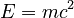$E=mc^2$, and is one of the most well known equations. In simple terms, if you take the mass of something and then multiply it by the speed of light, and then multiply it by the speed of light again, you will get how much pure energy a given piece of something has. Since the speed of light is such a big number, this means that even a small amount of matter can have a lot of energy (it has been projected to be 4 times more effective per mass than nuclear fission).

In 1928, the physicist Paul Dirac was looking for an equation that would predict how very fast particles should behave. There was already another equation that could describe slow-moving particles, the Schrödinger equation, but Einstein's theory of special relativity said that fast particles could be very different from slow particles. Dirac knew that particles like electrons were usually moving very fast. He realized the old equation would not make good predictions for fast particles. So he came up with a new equation that could describe particles moving close to the speed of light.

For fast particles, it is no longer true that the energy is$E=mc^2$. Instead, Dirac's new equation worked for particles where the energy was given by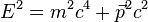$E^2=m^2c^4 + \vec{p}^2 c^2$. In the new equation for energy, the symbol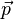$\vec{p}$is called the momentum, and it measures how fast the particle is going and how hard it is to stop. This equation says that very fast particles have more energy, so they are different from slow particles. You can take the square root of each side of this equation, since both sides are equal. However, any real square root has two answers,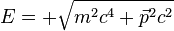$E=+\sqrt{m^2c^4 + \vec{p}^2 c^2}$and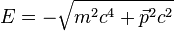$E=-\sqrt{m^2c^4 + \vec{p}^2 c^2}$. You can think of the answer with negative energy as being antimatter.

The reason this is important to understand antimatter is because scientists found that when matter and antimatter touch each other, the amount of energy that is released comes very close to the amount of energy$E=mc^2$ says should be all together in those two pieces. The reason is that each particle of matter, when it touches its antiparticle in the antimatter world, both change over into pure energy, or annihilate each other. This release of such a high amount of energy is why a lot of science fiction writers use antimatter for fuel in their stories. For example, author Dan Brown uses antimatter in "Angels and Demons" as a very powerful weapon. It is also being looked at as a fuel source for real-life missions to outer space in the future.

## Where is all the antimatter?

Many scientists think that in the first few moments after the Big Bang, which created the universe a very long time ago, both matter and antimatter mixed together. If the Big Bang made equal amounts of matter and antimatter, then the two would annihilate and become energy. After a long time, there would be no matter and no antimatter left, just energy. But our universe today looks like it is almost all matter and hardly any antimatter. Physicists do not yet know for sure that equal amounts of matter and antimatter were created, and because of this, they are also wondering where the antimatter went, and if any was left over from the beginning of the universe.

One explanation is that there was just a little bit more matter than antimatter in the beginning, so that whatever was left over after most of the matter and antimatter annihilated into energy became the mostly-matter universe we see today. Another theory is that there is lots of antimatter on the other side of the universe, hidden far beyond our vision. They could have formed their own galaxies and solar systems too.

## Uses

Because antimatter can make so much energy, it can be used for a lot of things, such as fuel for going into outer space, or in our cars. The problem is that antimatter is very expensive to make, and is almost as expensive to store, since it cannot touch regular matter. It takes several hundred million dollars to make less than one-millionth of a gram of antimatter. In fact, it is the most expensive and the rarest substance on Earth. Since it is so expensive, this means that antimatter is not practical to use as a weapon or as an energy source, because so little of it is obtainable.

Recently, however, scientists have trapped antimatter for over 16 minutes (1000 sec. total).

It does have a use in medicine, because a special kind of scanner called the PET, which stands for positron emission tomography, uses positrons to go into the human body. Doctors can look at the way the positron changes into energy inside a person's body, and be able to tell if something is wrong inside a person. This kind of machine works differently than an X-ray machine or a magnetic resonance imaging (MRI) machine, and can help doctors see things that those other machines cannot see.Antimatter Facts for Kids. Kiddle Encyclopedia.